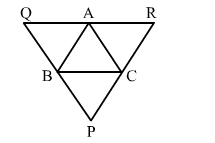# A ∆ABC is given. If lines are drawn through A, B, C, parallel respectively to the sides

Question:

A $\triangle A B C$ is given. If lines are drawn through $A, B, C$, parallel respectively to the sides $B C, C A$ and $A B$, forming $\triangle P Q R$, as shown in the adjoining figure, show that $B C=\frac{1}{2} Q R$.Solution:

BC || QA and CA || QB
i.e., BCQA is a parallelogram.
∴ BC = QA                           ...(i)
Similarly, BC || AR and AB || CR.
i.e., BCRA is a parallelogram.
∴ BC = AR                         ...(ii)
But QR = QA + AR
From (i) and (ii), we get:
QR = BC + BC
⇒ QR = 2BC

$\therefore B C=\frac{1}{2} Q R$